# Visual Reasoning Questions

03 September 2023

## Visual Reasoning Questions With Answers

### Q1) Observe the problem figures given and select one option from the answer figures which will continue the same pattern followed in the problem figures.

1. 1

#### Problem figures: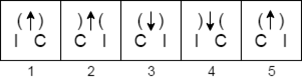A.         5

B.         4

C.         3

D.         2

1.2)

#### Problem figures;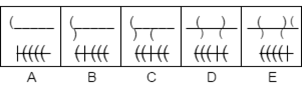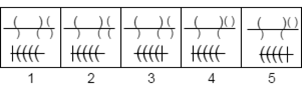A.         2

B.         1

C.         3

D.         5

1.3)

#### Problem figures;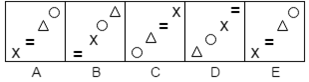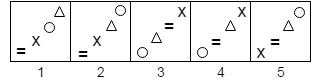A.         1

B.         3

C.         5

D.         4

1.4)

#### Problem figures;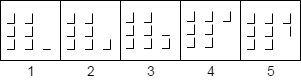A.         1

B.         2

C.         3

D.         4

1.5)

#### Problem figures;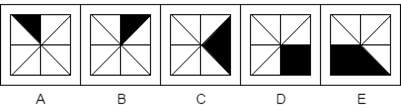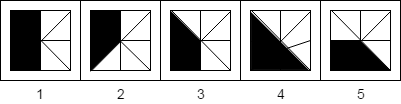A.         3

B.         5

C.         1

D.         2

### 2) In the below question one main figure is embedded in any of the four option figures. Find the option which contains main figure.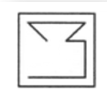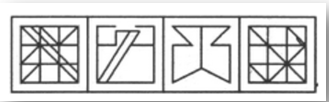A.         3

B.         4

C.         1

D.         2

### 3) In the below question one main figure is embedded in any of the four option figures. Find the option which contains main figure.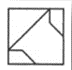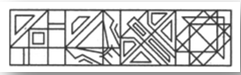A.         3

B.         4

C.         1

D.         2

### 8) Observe the problem figures given and select one option from the answer figures which will continue the same pattern followed in the problem figures.

8.1)

#### Problem figures;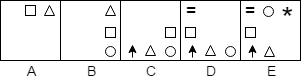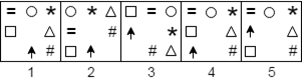A.         4

B.         5

C.         3

D.         1

8.2)

#### Problem figures;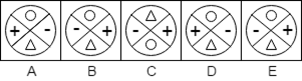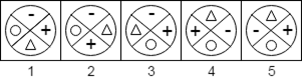A.         2

B.         4

C.         5

D.         1

8.3)

#### Problem figures;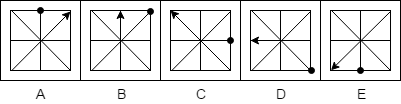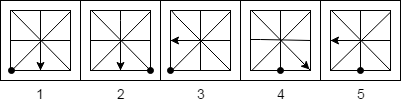A.         4

B.         3

C.         2

D.         1

8.4)

#### Problem figures;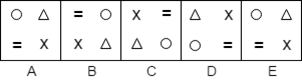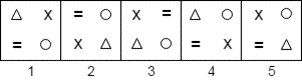A.         2

B.         3

C.         4

D.         5

8.5)

#### Problem figures;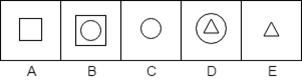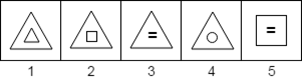A.         5

B.         3

C.         4

D.         2

8.6

#### Problem figures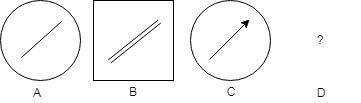A.         4

B.         1

C.         3

D.         2

##### Explanation: In the series of problems figures, a circle alternates with square, and a single line alternates with single line arrow. So, the answer figure 4 should complete the problem series, as it is a square and also contains a double line arrow.

9) Find out which one of the 4 figures is the missing part in the main figure.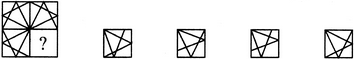(1)                 (2)                 (3)                 (4)

A.         1

B.         2

C.         3

D.         4

### 10) Find out which one of the 4 figures is the missing part in the main figure.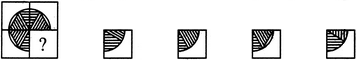(1)                 (2)                 (3)                 (4)

A.         1

B.         2

C.         3

D.         4

### 11. Find out which one of the 4 figures is the missing part in the main figure.(1)                 (2)                 (3)                 (4)

A.         4

B.         3

C.         2

D.         1

### 12. Find out which one of the 4 figures is the missing part in the main figure.(1)                           (2)                            (3)                            (4)

A.         1

B.         2

C.         3

D.         4

### 13. In the below question the problem figures follow a specific series is followed in the answer figures. Find the option which maintains the correct next series.

#### Problem Figures: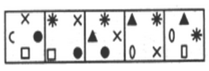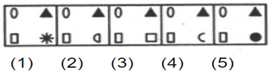A.         1

B.         2

C.         3

D.         4

### 14. Choose the correct water - image of the main figure from the given four figures.

#### Main Figure: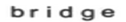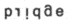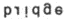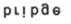A.         4

B.         3

C.         2

D.         1

##### Explanation: As per the rules of water image the reflection of an object into the water is its water image. It appears by inverting an object vertically i.e., upside down. Hence, option 2 is the reflection among all.

15) Observe the problem figures given and select one option from the answer figures which will continue the same pattern followed in the problem figures.

#### 15.1)      Problem figures                                Answer figures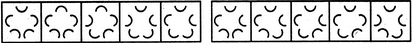A.         4

B.         3

C.         2

D.         1

15.2)

#### Answer Figures: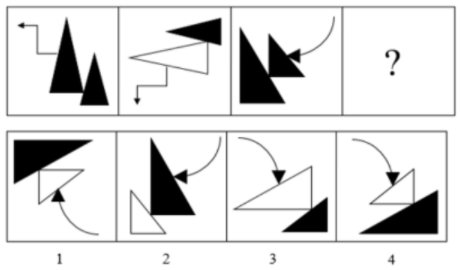A.         3

B.         2

C.         4

D.         1

##### Explanation: The pattern is, the 2nd box is the 90 degree Anti-Clock Wise rotation of the 1st box and on of the two shaded triangles is non shaded. Only 4 in the answer figure, follows the same pattern.

15.3)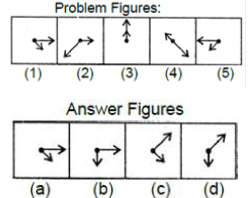A.         3

B.         2

C.         4

D.         1

15.4)

#### Problem Figure: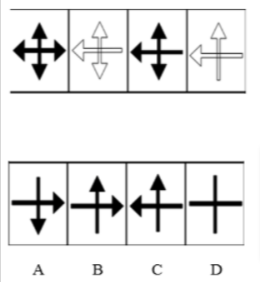(1)           (2)              (3)            (4)

A.         3

B.         2

C.         4

D.         1

15.5)

#### Problem Figure: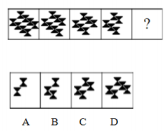A.            1

B.            2

C.            3

D.            4

### 16) Choose the correct water - image of the main figure from the given four figures.

#### Main Figure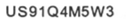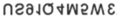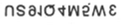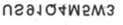A.            1

B.            2

C.            3

D.            4